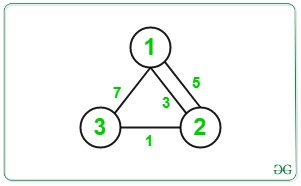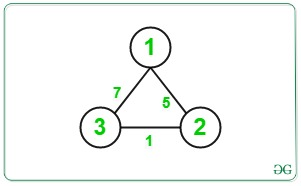# Minimum Cost using Dijkstra by reducing cost of an Edge

Given an undirected graph of N nodes and M edges of the form {X, Y, Z} such that there is an edge between X and Y with cost Z. The task is to find the minimum cost to traverse from source node 1 to destination node N such we are allowed to reduce the cost of only one path during traversal by 2.

Examples:

Input: N = 3, M = 4, Edges = {{1, 2, 3}, {2, 3, 1}, {1, 3, 7}, {2, 1, 5}}
Output: 2
Explanation:Minimum Cost from source node 1 to destination node N is = 3/2 + 1 = 1 + 1 = 2.

Input: N = 3, M = 3, Edges = {{2, 3, 1}, {1, 3, 7}, {2, 1, 5}}
Output: 2
Explanation:Minimum Cost from source node 1 to destination node N is = 7/2 = 3.

## Recommended: Please try your approach on {IDE} first, before moving on to the solution.

Approach: The idea is to consider every edge and try to minimize the overall cost by reducing its cost. The main idea is to break the path between source to destination into the source to any vertex u i.e., path(1 to u) and from destination to any vertex v i.e., path(n to v) for all u and v. Below are the steps:

1. Perform a Dijkstra Algorithm to find the single source shortest path for all the vertex from source node 1 and store it in an array as dist_from_source[].
2. Perform a Dijkstra Algorithm to find the single source shortest path for all the vertex from source node N and store it in an array as dist_from_dest[].
3. Initialise the minimum cost(say minCost) as maximum value.
4. Traverse the given edges and for each edges reduce the current cost to half and update the minimum cost as:

minCost = min(minCost, dist_from_source[u] + c/2 + dist_from_dest[v])
where,
c is the cost of current edge,
dist_from_source[u] is cost of path from node 1 to u
dist_from_source[v] is cost of path from node v to N

5. Print the value of minCost after the above step.

Below is the implementation of the above approach:

## C++14

 `// C++ program for the above approach ` `#include ` `using` `namespace` `std; ` `#define INF 1e9 ` ` `  `// Function for Dijkstra Algorithm to ` `// find single source shortest path ` `void` `dijkstra(``int` `source, ``int` `n, ` `              ``vector > ` `                  ``adj[], ` `              ``vector<``int``>& dist) ` `{ ` `    ``// Resize dist[] to N and assign ` `    ``// any large value to it ` `    ``dist.resize(n, INF); ` ` `  `    ``// Initialise distance of source ` `    ``// node as 0 ` `    ``dist = 0; ` ` `  `    ``// Using min-heap priority_queue ` `    ``// for sorting wrt edges_cost ` `    ``priority_queue, ` `                   ``vector >, ` `                   ``greater > > ` `        ``pq; ` ` `  `    ``// Push the current dist ` `    ``// and source to pq ` `    ``pq.push({ dist, source }); ` ` `  `    ``// Until priority queue is empty ` `    ``while` `(!pq.empty()) { ` ` `  `        ``// Store the cost of linked ` `        ``// node to edges ` `        ``int` `u = pq.top().second; ` `        ``// int d = pq.top().first; ` ` `  `        ``// Pop the top node ` `        ``pq.pop(); ` ` `  `        ``// Iterate over edges ` `        ``for` `(``auto``& edge : adj[u]) { ` ` `  `            ``// Find the starting and ` `            ``// ending vertex of edge ` `            ``int` `v = edge.first; ` `            ``int` `w = edge.second; ` ` `  `            ``// Update the distance of ` `            ``// node v to minimum of ` `            ``// dist[u] + w if it is ` `            ``// minimum ` `            ``if` `(dist[u] + w < dist[v]) { ` `                ``dist[v] = dist[u] + w; ` `                ``pq.push({ dist[v], v }); ` `            ``} ` `        ``} ` `    ``} ` `} ` ` `  `// Function to find the minimum cost ` `// between node 1 to node n ` `void` `minCostPath( ` `    ``vector > >& edges, ` `    ``int` `n, ``int` `M) ` `{ ` ` `  `    ``// To create Adjacency List ` `    ``vector > adj; ` ` `  `    ``// Iterate over edges ` `    ``for` `(``int` `i = 0; i < M; i++) { ` ` `  `        ``// Get source, destination and ` `        ``// edges of edges[i] ` `        ``int` `x = edges[i].first; ` `        ``int` `y = edges[i].second.first; ` `        ``int` `z = edges[i].second.second; ` ` `  `        ``// Create Adjacency List ` `        ``adj[x].push_back({ y, z }); ` `        ``adj[y].push_back({ x, z }); ` `    ``} ` ` `  `    ``// To store the cost from node 1 ` `    ``// and node N ` `    ``vector<``int``> dist_from_source; ` `    ``vector<``int``> dist_from_dest; ` ` `  `    ``// Find the cost of travel between ` `    ``// source(1) to any vertex ` `    ``dijkstra(1, n + 1, adj, dist_from_source); ` ` `  `    ``// Find the cost of travel between ` `    ``// destination(n) to any vertex ` `    ``dijkstra(n, n + 1, adj, dist_from_dest); ` ` `  `    ``// Initialise the minimum cost ` `    ``int` `min_cost = dist_from_source[n]; ` ` `  `    ``// Traverse the edges ` `    ``for` `(``auto``& it : edges) { ` ` `  `        ``// Get the edges ` `        ``int` `u = it.first; ` `        ``int` `v = it.second.first; ` `        ``int` `c = it.second.second; ` ` `  `        ``// Find the current cost from ` `        ``// node 1 to u and node u to v ` `        ``// and node v to N with only ` `        ``// current edge cost reduced ` `        ``// to half ` `        ``int` `cur_cost = dist_from_source[u] ` `                       ``+ c / 2 ` `                       ``+ dist_from_dest[v]; ` ` `  `        ``// Update the min_cost ` `        ``min_cost = min(min_cost, cur_cost); ` `    ``} ` ` `  `    ``// Print the minimum cost ` `    ``cout << min_cost << ``'\n'``; ` `} ` ` `  `// Driver Code ` `int` `main() ` `{ ` `    ``// Give Nodes and Edges ` `    ``int` `N = 3; ` `    ``int` `M = 3; ` ` `  `    ``// Given Edges with cost ` `    ``vector > > edges; ` ` `  `    ``edges.push_back({ 2, { 3, 1 } }); ` `    ``edges.push_back({ 1, { 3, 7 } }); ` `    ``edges.push_back({ 2, { 1, 5 } }); ` ` `  `    ``// Function Call ` `    ``minCostPath(edges, N, M); ` `    ``return` `0; ` `} `

Output:

```3
```

Time Complexity: O(N + M), where N is the number of nodes and M is the number of edges.
Auxiliary Space: O(N), where N is the number of nodes.

Attention reader! Don’t stop learning now. Get hold of all the important DSA concepts with the DSA Self Paced Course at a student-friendly price and become industry ready.

My Personal Notes arrow_drop_upCheck out this Author's contributed articles.

If you like GeeksforGeeks and would like to contribute, you can also write an article using contribute.geeksforgeeks.org or mail your article to contribute@geeksforgeeks.org. See your article appearing on the GeeksforGeeks main page and help other Geeks.

Please Improve this article if you find anything incorrect by clicking on the "Improve Article" button below.